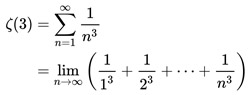A# Apéry's constantRoger Apéry.

Apéry's constant is defined as:where ζ is the Riemann zeta function.

It has the approximate value 1.202056903...

It also arises in the following way: pick three positive integers at random, then the odds of them having no common divisor are 1 in 1.202056903...

In 1979, the French mathematician Roger Apéry (1916–1994) stunned the mathematical world with a proof that this number is irrational.1 Whether it is a transcendental number remains an open question.

### Reference

1. Apéry, R. "Irrationalité de ζ(2) et ζ(3)." Astérisque, 61: 11-13 (1979).